# Cutting Up a Circle I: Using n Chords

We’ve looked at how to count diagonals in a polygon; this week and next, I want to consider two different problems (though they look similar at first) dealing with chords of a circle (which are practically the same thing as diagonals of a polygon). In each, what we count will be the regions into which the circle is cut. We’ll see how several Math Doctors solved the problem, each from a sufficiently different perspective that it might communicate better to certain readers; so if you don’t follow one, keep reading!

For the first problem, we can start with this question from 1998:

Regions, Chords, and Circles

A given circle has n chords. Each chord crosses every other chord but no three meet at the same point. How many individual regions are in the circle?

This is how much I've completed on this problem:  I know that the xth line splits x regions, increasing the number of sections by x. I'm having trouble finding out the formula to solve the problem.

Please help me!

Here is a simple example, with 3 chords dividing the circle into 7 regions: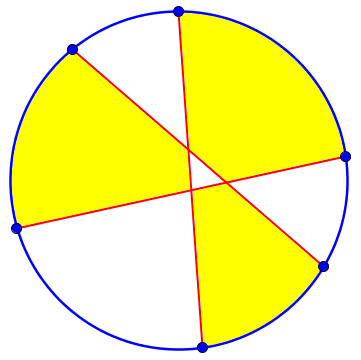We’ll be seeing different characterizations of the same problem, in which we are only asked for the maximum number of regions; that will turn out to imply this idea that each chord intersects all the others. For example, if my three chords intersected at one point, we would lose a region, and have only six: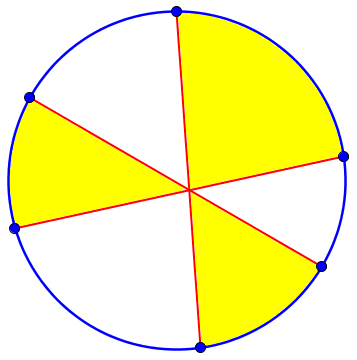On the other hand, if any chord does not intersect all the others, we again have fewer than 7 regions: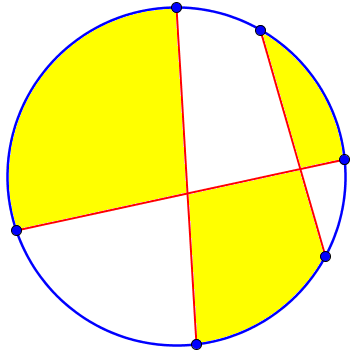Doctor Jaffee replied, using an approach based on looking for a pattern in the numbers:

Hi Dana,

Your observation about how the number of regions increases with each additional chord is right on target. We can use that to construct a table of values that can be helpful:

number of chords        1   2   3   4   5   6
number of regions       2   4   7  11  16  22

You noticed that the number of regions increases by 2, then 3, then 4, etc., and that is one of the properties of quadratic functions; that is, as the x number increases by 1, the y number increases by a constantly increasing amount. In the example above each increase is 1 more than the previous increase.



Here are the first few cases, showing how each new chord n crosses each of the previous n – 1 chords, cutting off n new regions (one from each of the n regions it passed through), thereby adding 1, then 2, then 3, then 4 regions: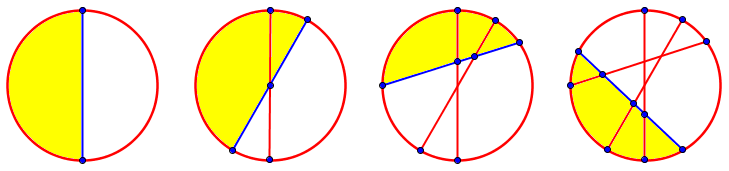Doctor Jaffee has made a table of values, then used the fact that if the difference increases by the same amount at each step, the formula will have quadratic form. For example, looking at the perfect squares, 1, 4, 9, 16, we see that the differences from one term to the next are 3, 5, 7, and so on, always increasing by 2.

So, we can pick any three ordered pairs from the chart, substitute the values into the standard equation of a quadratic function (y = ax^2 + bx + c). We will then have three equations in three variables which we can solve. Substitute those numbers back into the standard equation and we'll have finished.

We’re going to solve for the three coefficients of the formula.

It looks like this:

I would pick the first three pairs (1,2), (2,4), (3,7). Substitute them into the standard quadratic form and get

2 =  a +  b + c
4 = 4a + 2b + c
7 = 9a + 3b + c

The solution to this system is a = 1/2,  b = 1/2,  and c = 1.

So, substituting them into y = ax^2 + bx + c we get

y = (1/2)x^2 + (1/2)x + 1

where x is the number of chords and y is the number of regions formed.  You can then verify that if x = 4, y turns out to be 11 just as in the chart. If x = 5, y = 16, and so on.

One way to solve the system of equations is to first subtract each equation from the next one, leaving two equations in two unknowns: $$2=3a+b\\3=5a+b$$

Then we can subtract the first of these two from the second: $$1=2a$$ so that $$a=\frac{1}{2}$$, and then, putting that into the first of the two equations, $$2=3\cdot\frac{1}{2}+b$$, so $$b=\frac{1}{2}$$; and then from the first original equation, $$2=\frac{1}{2}+\frac{1}{2}+c$$, and $$c=1$$.

In the terms I’ll be using, taking n = number of chords and r = number of regions, the formula is $$r = \frac{1}{2}n^2 + \frac{1}{2}n + 1 = \frac{n^2+n+2}{2}$$

## Drawing six chords

Dividing a Circle using Six Lines

What is the largest number of regions into which you can divide a circle with six lines? Is there a formula? How can you get this answer? I've tried drawing diagrams but I can't find a way to make sure they are correct. I can only get 14, but I know there are more.

Here the problem is phrased in terms of the maximum number of regions, rather than telling us each chord crosses all the others; and it asks about a specific number of chords, not a formula. But a formula can help Jordy know whether what he has drawn is the best he can do. And so will the concepts behind the formula.

Doctor Rob was the first to answer, providing the formula we just saw (without proof) and a way to actually draw the figure, which accords with our observations above:

Thanks for writing to Ask Dr. Math, Jordy.

There is a formula.  For n lines, you can get at most

(n^2+n+2)/2

regions.  When n = 6, this gives 22 regions.



Here is the benefit of having a formula; we can tell whether we have reached our goal in the drawing.

To maximize the number of regions, draw your lines like this:

Start with one line. Label the two points where it meets the circle as P1 and Q1.

First chord:Pick points P2 and Q2 such that they are encountered in the order P1,P2,Q1,Q2,P1 going around the circle clockwise. Connect P2 and Q2 to make the second line, and four regions.

Second chord: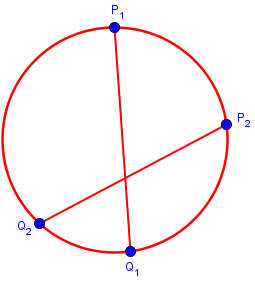If the new points were on the same side of the first chord, we wouldn’t get the maximum number of regions, so the order is important.

Between P2 and Q1 pick point P3. Between Q2 and P1 pick point Q3.  Connect them with a line, but, if necessary, move Q3 a little to make sure that the line does not pass through any of the intersections of any previously drawn lines. That will give you seven regions.

Third chord: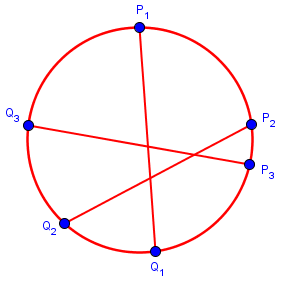Again, we have to cross both existing chords, so it has to start between the last P and the first Q, and end between the last Q and the first P.

Between P3 and Q1 pick point P4. Between Q3 and P1 pick point Q4. Connect P4 to Q4 with a line, but, if necessary, move Q4 a little to make sure that the line does not pass through any of the intersections of any previously drawn lines. That will give you 11 regions.

Fourth chord: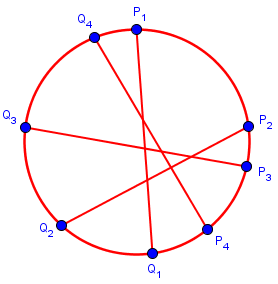It’s getting harder to avoid intersections, as the regions are getting small.

Continue in this way until you have 6 lines and 22 regions.

Here is my result, after making many adjustments of existing points to make room: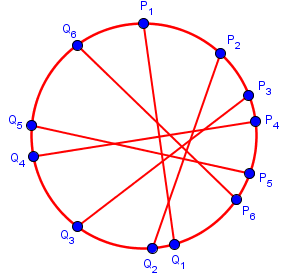Now he suggests a more orderly way to pick the points that will ensure that everything fits:

P1-P6 and Q1-Q6 can be chosen to be 12 adjacent vertices of a regular n-gon inscribed in the circle, if n > 12. It is easy to construct a regular 16-gon by starting with a diameter of the circle and bisecting the central angles until they are all equal to 360/16 = 22.5 degrees.  That will locate 16 equally-spaced points around the circumference of the circle. Pick any 12 that are adjacent, and label them P1, P2, ..., P6, Q1, ..., Q6. Then connect P1Q1, P2Q2, ..., P6Q6. If you have done this carefully, you will be able to see and count the 22 regions.

This produces a more regularly spaced pattern that is easier to count: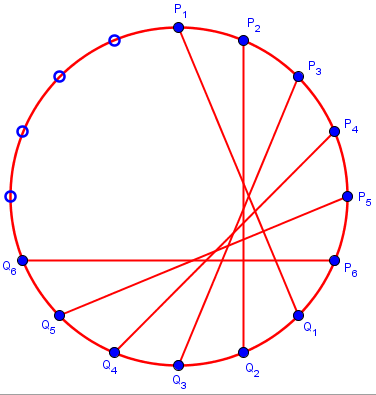## Counting step by step

Doctor Greenie, who probably had been writing as Doctor Rob wrote, stepped in with essentially the same  approach, but this time leading to the formula:

Hi, Jordy -

There is a formula - as I see another doctor here has already shown you.

But there is a way you can get the answer without the formula, by logically analyzing what happens as you draw more and more lines.  And, if you know the correct mathematical techniques, this logical analysis can lead you to a derivation of the formula.

I have always found mathematics to be more enjoyable when I can see where a formula comes from, rather than having to regard it as some sort of mathematical magic.

So here is how you can analyze this problem, at least far enough to come up with the answer to your problem.

You are going to analyze how and when the number of regions increases as you draw more lines through the circle. You will find that, if you think of actually physically drawing each line, the number of regions increases by one at a time; each increase is the result of an existing region being cut into two separate regions.

Start with a circle; before you draw any line through the circle, it is a single region.

If you’ve followed the thinking as others showed it, you may skip the details below; but if you need any help being sure of the hows and whys, this should make it clear. If I drew pictures for this, they would be the same we’ve already seen:Now, start drawing the first line through the circle, and consider at what point(s) you add another region to the diagram. When you are almost all the way from your starting point to the other side of the circle, there is still only one region; it is not until you reach the other side that you have divided an existing region (in this case, the entire circle) into two parts. So, when you draw the first line through the circle, there is one point at which one of the existing regions becomes two regions: when you reach the opposite side of the circle. So we have:

1st line drawn through circle ==> number of additional regions = 1

Next, start drawing the second line through the circle, and consider at what point(s) you add another region to the diagram. Of course, since you want to create the largest number of regions in the circle, you want your second line to intersect the first line inside the circle. So you start at some point on the circle, and when you reach the first line somewhere inside the circle you have added another region by dividing an existing region into two parts. And then when you reach the other side of the circle you have again added another region by dividing an existing region into two parts. So, when you draw the second line through the circle, there are two points at which one of the existing regions becomes two regions: when you reach the first line you drew, and when you reach the opposite side of the circle. So we have:

2nd line drawn through circle ==> number of additional regions = 2

Now, start drawing the third line through the circle, and consider at what point(s) you add another region to the diagram. Of course, since you want to create the largest number of regions in the circle, you want this third line to intersect both of the first two lines at different points inside the circle (if your third line intersects the first two lines at their point of intersection, you don't get the maximum possible number of regions). So you start at some point on the circle, and when you reach the first line somewhere inside the circle you have added another region by dividing an existing region into two parts, and when you reach the second line you have again added another region. And when you reach the other side of the circle you have again added another region by dividing an existing region into two parts.  So, when you draw the third line through the circle, there are three points at which one of the existing regions becomes two regions: when you reach each of the the first two lines you drew, and when you reach the opposite side of the circle. So we have:

3rd line drawn through circle ==> number of additional regions = 3

Now we generalize:

I think you can see where the analysis will go from here....

When you draw the n-th line, you create n new regions -- (n-1) new regions, one for each time you intersect one of the previous (n-1) lines that you drew, and a last time when you reach the other side of the circle.

So you can answer your specific question by making a simple table

number of   number of   total number
lines    new regions   of regions
--------------------------------------
0            --            1
1             1            2
2             2            4
3             3            7
4             4           11
5             5           16
6             6           22

So rather than actually drawing all the way to 6 lines, we can quickly make a table, and get to that answer of 22. Or, we can turn this into a formula:

Then there are various mathematical techniques (which I won't go into here) for examining the sequence of the number of regions

1, 2, 4, 7, 11, 16, 22, ...

to come up with the formula for the maximum number of regions formed by n lines:

n^2+n+2
# regions with n lines = ---------
2

One way to do this is to see that the total number of regions is $$1 + (1 + 2 + 3 + … + n)$$, and there is a formula for the sum from 1 to n (called the nth triangular number), namely $$1+2+3+…+n = \frac{n(n+1)}{2}$$ In fact, this is the same idea we used in counting handshakes. So our formula becomes $$r = 1 + (1 + 2 + 3 + … + n) = 1 + \frac{n(n+1)}{2} = \frac{n(n+1)+2}{2} = \frac{n^2+n+2}{2}$$ as before.

## From recursion to summation to formula

For one more derivation of the formula, we turn to this question from 2001:

Circle Regions

What is the maximum number of regions you can have with n chords in a circle?

With 0 chords you have 1 field
With 1 chord you have  2 fields; 1 more
2                 4         2 more
3                 7         3
4                11         4
etc.

Now I need a formula and a proof why this is so. Can you help me?

Here the anonymous questioner has already made the table and seen the recursive description, and wants a formula. Doctor Anthony replied, first writing the recursion explicitly:

We can find a general formula for the number of regions when the interior of a circle is divided by n lines.

Suppose the number of regions is given by f(n) when there are n lines drawn in the circle. Now draw one more line cutting all the other n lines. There are n points on the additional line, and so this line must traverse n+1 of the available f(n) regions, dividing each into two parts. It therefore adds n+1 more regions to those present. Thus

f(n+1) = f(n) + n+1

As we’ve seen, each added line adds as many regions as the new number of lines. This could have been written as $$f(n)=f(n-1)+n$$, but here we are thinking of obtaining the next number when we already have $$f(n)$$. Now he writes this as a “telescoping” sequence of equations:

We can write  f(n+1) - f(n) = n+1  and now form a succession of equations as follows:

f(1) - f(0) =  1
f(2) - f(1) =  2
f(3) - f(2) =  3
...................
...................
f(n-1) - f(n-2) =  n-1
f(n)   - f(n-1) =  n
f(n) - f(0)  = SUM(1 to n)   (note cancellation between lines)

f(n) - 1  = n(n+1)/2

f(n) = n(n+1)/2  + 1

This is equivalent to what I said at the end of Doctor Greenie’s method.

Check if this is correct  n=0  gives  f(0) = 1
n=1  gives  f(1) = 2
n=2  gives  f(2) = 4  and these are correct.

With n = 4 we get   4 x 5/2 + 1  =  11  regions.

And so on.

Checking a few known answers is a good practice whenever you obtain a general formula!

Next week: What if we are given n points on the circle, and connect them with all possible chords?

### 5 thoughts on “Cutting Up a Circle I: Using n Chords”

1.I understand that to see a quadratic pattern we have to consider 3 points.
But I did not understand the following ,
“that is one of the properties of quadratic functions; that is, as the x number increases by 1, the y number increases by a constantly increasing amount.”
I calculated following, that is increase in y value
R2-R1=3a+b,
R3-R2=5a+b,
R4-R3=7a+b etc.
Does the above statement mean that I have to again subtract these from each other so that I get a constant difference of 2a?
Difference of the differences, Increasing by a constant amount hence the amount is Constantly increasing .
Regards,
Rahul.

2.Yes.
I got it.
The first differences are not constant.
Those are in fact 3a+b, 5a+b, 7a+b etc.
While the second differences are constant i.e. 2a.
This tells me that the pattern must be quadratic.
Is this sort of a rule?
Had the third differences been constant instead of second?, the pattern would have been cubic?
Regards,
Rahul

1.Yes, you are right. If the third differences are constant (but not zero), the sequence can be represented by a cubic equation, and so on.

I plan to eventually post about this topic, but here is one of the central discussions on “finite differences” in Ask Dr. Math: Finding the Rule for a Given Sequence

The general case is discussed in Proof of Successive Differences and the Degree of a Polynomial and in Finite Differences and Polynomials

This site uses Akismet to reduce spam. Learn how your comment data is processed.